# 渗透测试之通过代码审计打点

1. 寻找敏感功能点，通读功能点代码；优点：精准定向挖掘，利用程度高；缺点：命名不规范的代码容易被忽略，导致失去先机。
2. 根据敏感关键字回溯参数传递过程；优点：通过搜索敏感关键字可快速定位可能存在的漏洞，可定向挖掘，高效、高质量；缺点：对程序整体架构了解不够深入，在漏洞定位时比较耗时，逻辑漏洞覆盖不到。
3. 直接通读全文代码；优点：对整体架构熟悉，了解程序的数据流处理、配置文件、过滤函数等；缺点：耗时长，需要足够的时间熟悉整体架构。

#### 案例一：一个任意文件下载漏洞引起的代码审计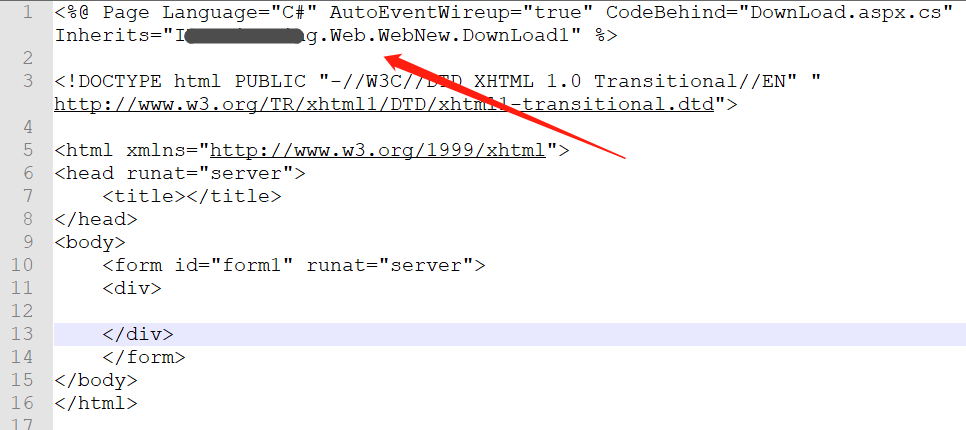Inherits是啥？

MSDN官方解释：定义供页继承的代码隐藏类。它可以是从 Page 类派生的任何类。此特性与 CodeFile 特性一起使用，后者包含指向代码隐藏类的源文件的路径。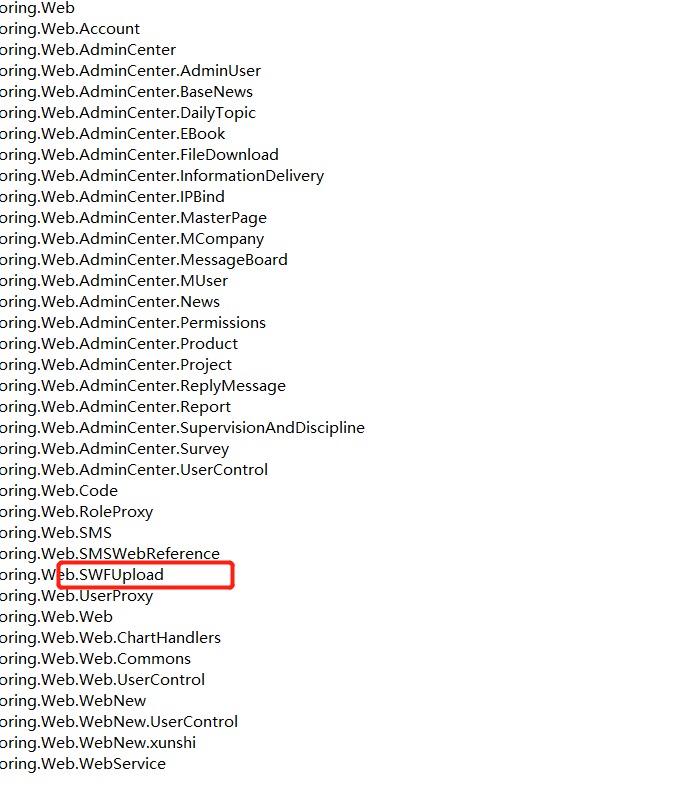````public void uploadFile()`
`        {`
`            string formStringParamValue = SWFUrlOper.GetFormStringParamValue("path");`
`            string formStringParamValue2 = SWFUrlOper.GetFormStringParamValue("fn");`
`            bool flag = SWFUrlOper.GetFormStringParamValue("small").ToLower() == "true";`
`            ……``            string[] array = new string[]`
`            {`
`                "jpg",`
`                "gif",`
`                "png",`
`                "bmp"`
`            };`
`            string formStringParamValue3 = SWFUrlOper.GetFormStringParamValue("data");`
`            try`
`            {`
`                System.Web.HttpPostedFile httpPostedFile = base.Request.Files["Filedata"];`
`                string b = string.Empty;`
`                string text = string.Empty;`
`                if (httpPostedFile.ContentLength > 0)`
`                {`
`                    text = httpPostedFile.FileName;`
`                    if (text.IndexOf(".") != -1)`
`                    {`
`                        b = text.Substring(text.LastIndexOf(".") + 1, text.Length - text.LastIndexOf(".") - 1).ToLower();`
`                    }`
`                    SWFUploadFile sWFUploadFile = new SWFUploadFile();`
`                    if (flag)`
`                    {`
`                        sWFUploadFile.set_SmallPic(true);`
`                        sWFUploadFile.set_MaxWith((formIntParamValue == 0) ? sWFUploadFile.get_MaxWith() : formIntParamValue);`
`                        sWFUploadFile.set_MaxHeight((formIntParamValue2 == 0) ? sWFUploadFile.get_MaxHeight() : formIntParamValue2);`
`                    }`
`                    sWFUploadFile.set_IsWaterMark(isWaterMark);``                    int num = 0;`
`                    string text2 = sWFUploadFile.SaveFile(httpPostedFile, formStringParamValue, formStringParamValue2, ref num);`
`                    ……`
`        }````

38行进行了文件保存，之前没有对文件的内容、后缀等有任何过滤，开开心心挖到任意文件上传。二话不说本地构造上传个shell。

````<form name="form" method="post" action="http://xxxx.com/cms/SWFUpload.aspx" enctype="multipart/form-data" >````
````<input type="file" name="Filedata">````
````<input type="submit" name="Submit" value="upload" ></form>````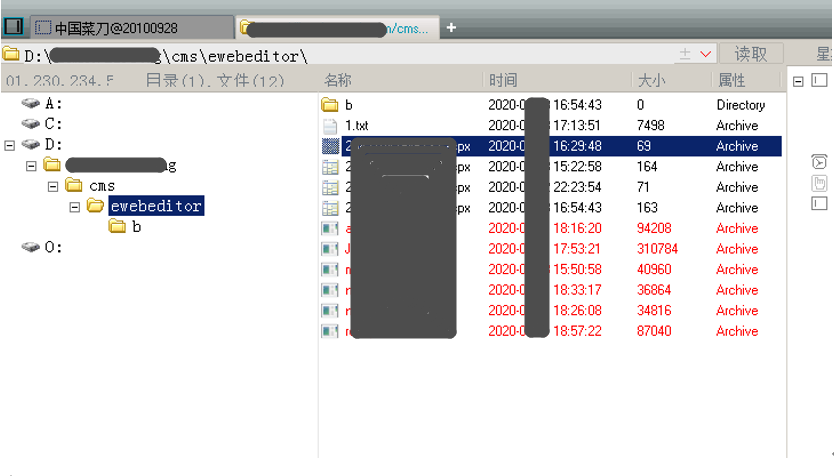````Dotfuscator`
`.NET Reactor`
`Xenocode`
`CryptoObfuscator`
`SmartAssembly`
`......````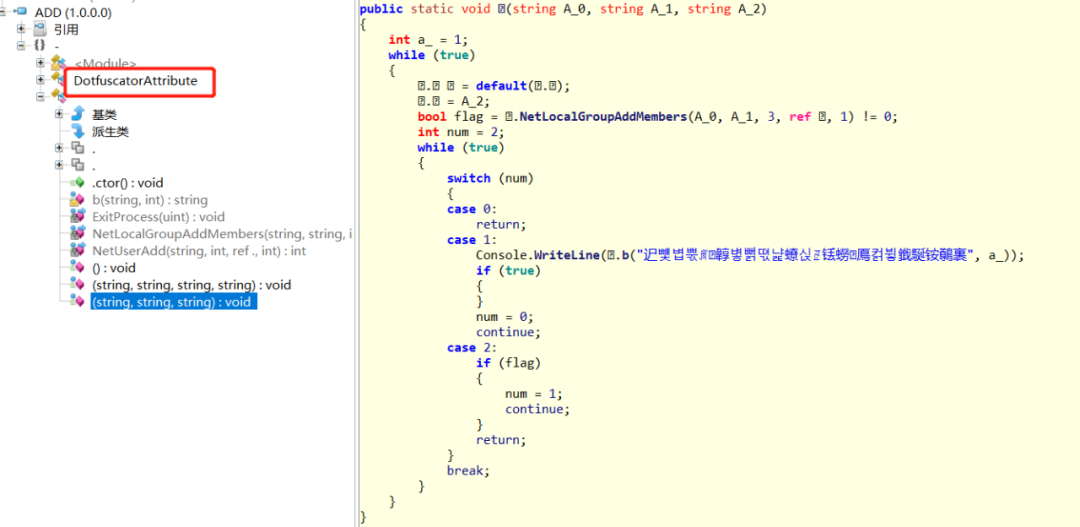`CMD命令行执行：de4dot.exe ADD.dll`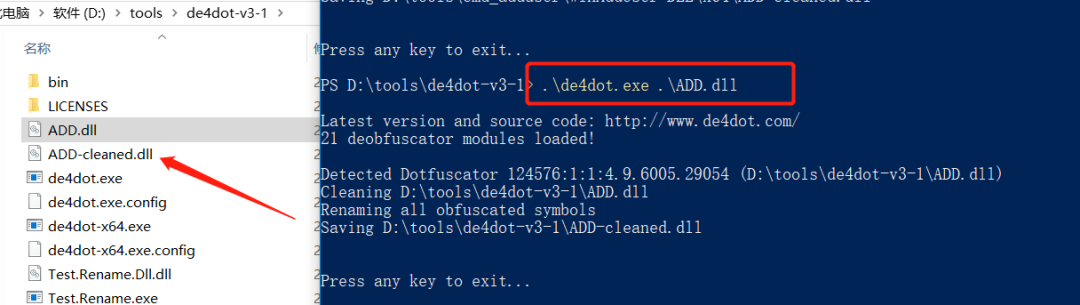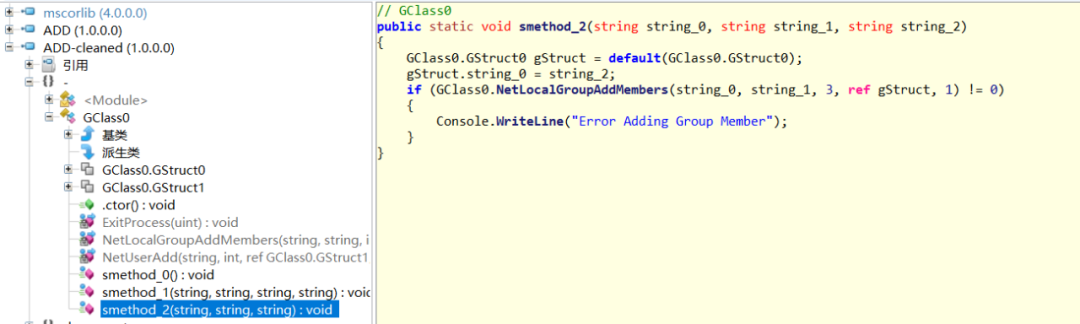#### 案例二：某个开源系统的代码审计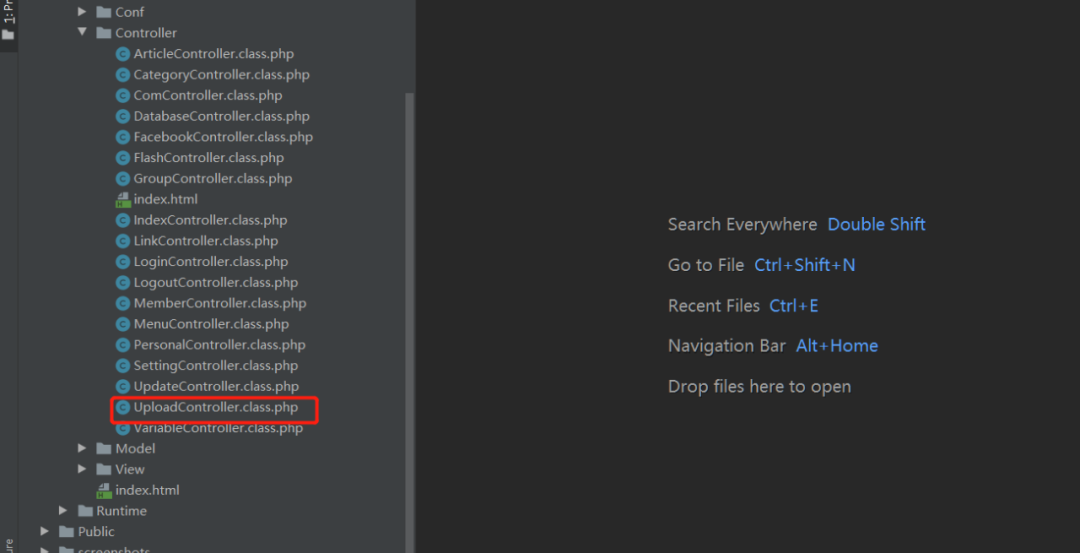````class UploadController extends ComController{`
` ......``private function saveimg(\$file){`
`        \$uptypes=array(`
`            'image/jpeg',`
`            'image/jpg',`
`            'image/jpeg',`
`            'image/png',`
`            'image/pjpeg',`
`            'image/gif',`
`            'image/bmp',`
`            'image/x-png'`
`        );`
`        \$max_file_size=2000000;     //上传文件大小限制, 单位BYTE`
`        \$destination_folder='Public/attached/'.date('Ym').'/'; //上传文件路径`
`        if(\$max_file_size < \$file["size"]){`
`            echo "文件太大!";`
`            return null;`
`        }`
`        if(!in_array(\$file["type"], \$uptypes)){`
`            echo "文件类型不符!".\$file["type"];`
`            return null;`
`        }`
`        if(!file_exists(\$destination_folder)){`
`            mkdir(\$destination_folder);`
`        }`
`        \$filename=\$file["tmp_name"];`
`        \$image_size = getimagesize(\$filename);`
`        \$pinfo=pathinfo(\$file["name"]);`
`        \$ftype=\$pinfo['extension'];`
`        \$destination = \$destination_folder.time().".".\$ftype;`
`        if (file_exists(\$destination) && \$overwrite != true){`
`            echo "同名文件已经存在了";`
`            return null;`
`        }`
`        if(!move_uploaded_file (\$filename, \$destination)) {`
`            return null;`
`        }`
`        return "/".\$destination;`
`    }`
`   ......````

````class ComController extends BaseController {`
`  public \$USER;`
`  public function _initialize(){`
`    C(setting());`
`    \$user = cookie('user');`
`    \$this->USER = \$user;`
`    \$url = U("login/index");`
`    if(!\$user){`
`      header("Location: {\$url}");`
`      exit(0);`
`    }``    \$Auth  =   new Auth();`
`    \$allow_controller_name=array('Upload');//放行控制器名称``    \$allow_action_name=array();//放行函数名称`
`    if(!\$Auth->check(CONTROLLER_NAME.'/'.ACTION_NAME,\$this->USER['uid'])&&!in_array(CONTROLLER_NAME,\$allow_controller_name)&&!in_array(ACTION_NAME,\$allow_action_name)){            `
`      \$this->error('没有权限访问本页面!');`
`    }````
````    \$user = member(intval(\$user['uid']));`
`    \$this->assign('user',\$user);`````命令执行 > 代码执行 > 文件上传 > 文件包含 > SQL注入 > 文件下载 > 逻辑漏洞 > SSRF > XSS ...`

de4dot项目地址:

https://github.com/0xd4d/de4dot/

• 回顶